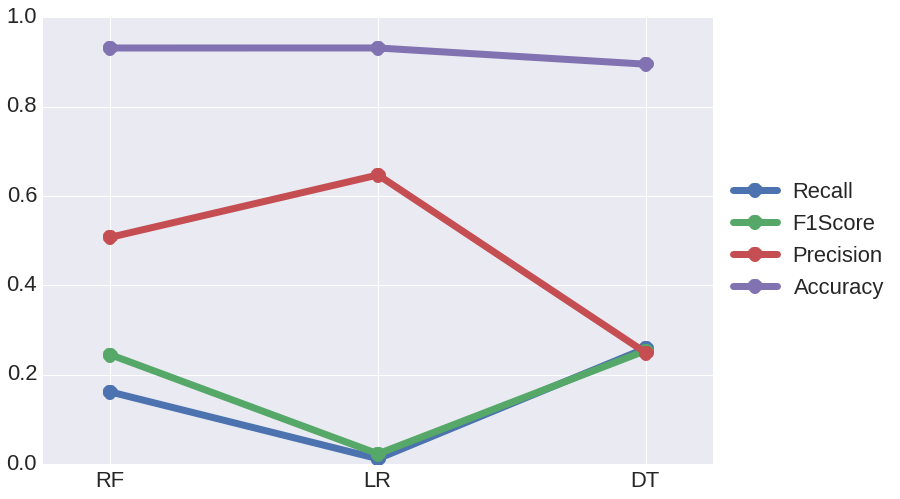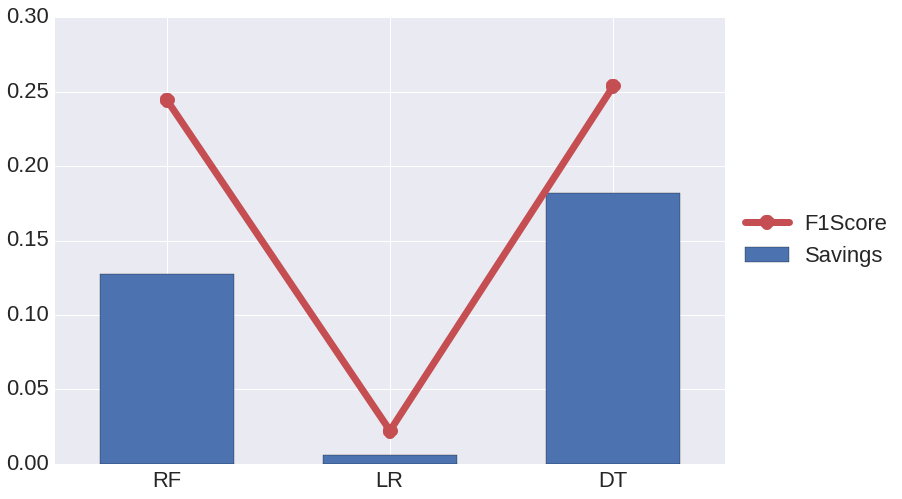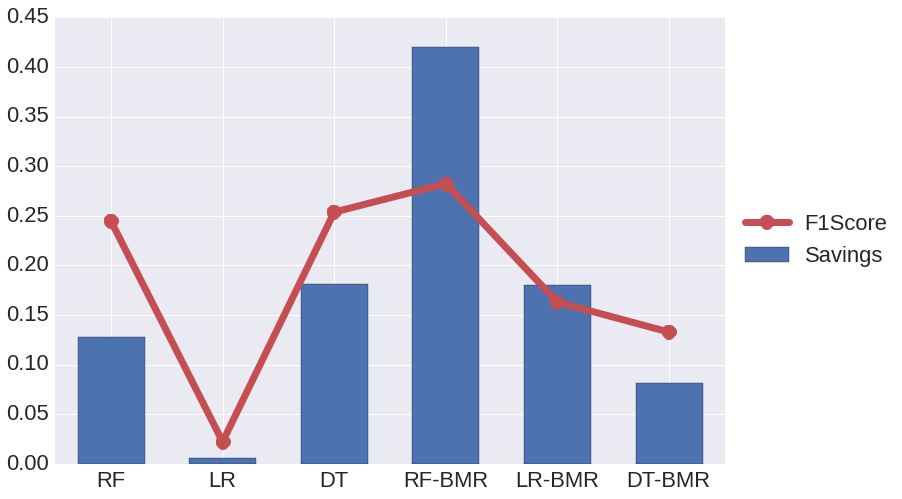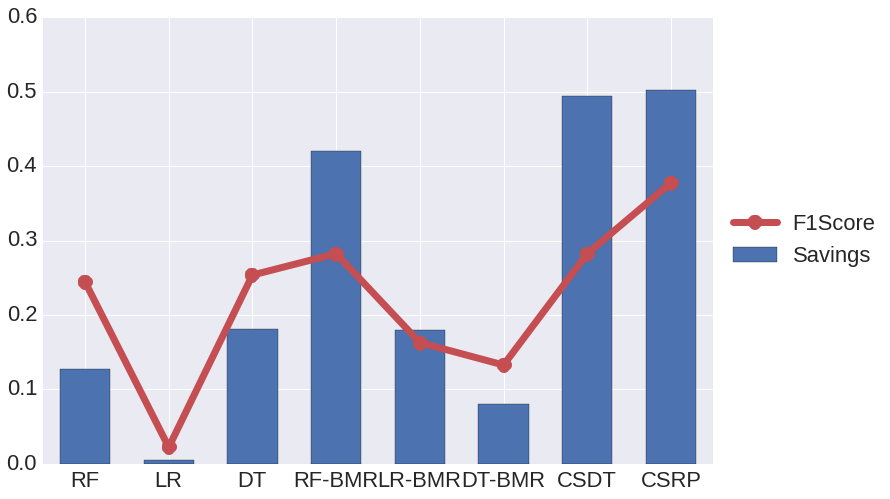Example-Dependent Cost-Sensitive Credit Scoring using CostCla

Alejandro Correa BahnsenA brief bio:¶

• Last year (month) PhD Student at Luxembourg University
• Work part time a fraud data scientist at CETREL a SIX Company
• Worked for +5 years as a data scientist at GE Money and Scotiabank
• Previously, six sigma intern at Dow Chemical
• Bachelor in Industrial Engineering and Master in Financial Engineering
• Organizer of Data Science Luxembourg and recently of Big Data Science Bog
• Sport addict, love to swim, play tennis, squash, and volleyball, among others.

Agenda¶

• Quick Intro to Credit Scoring
• Example of Credit Scoring
• Financial Evaluation of a Credit Scorecard
• Example-Dependent Classification
• CostCla Library
• Conclusion and Future Work

To whom would you grant a loan?¶Just fund a bank Just quit college

Nice guess!¶Biggest Ponzi scheme Now a Billionaire

Credit Scoring¶

• Mitigate the impact of credit risk and make more objective and accurate decisions
• Estimate the risk of a customer defaulting his contracted financial obligation if a loan is granted, based on past experiences
• Different machine learning methods are used in practice, and in the literature: logistic regression, neural networks, discriminant analysis, genetic programing, decision trees, random forests among others

Credit Scoring¶

Formally, a credit score is a statistical model that allows the estimation of the probability of a customer $i$ defaulting a contracted debt ($y_i=1$)

$$\hat p_i=P(y_i=1|\mathbf{x}_i)$$

Example: Kaggle Credit CompetitionImprove on the state of the art in credit scoring by predicting the probability that somebody will experience financial distress in the next two years.

https://www.kaggle.com/c/GiveMeSomeCredit

In :

Data file¶

In :
print data.keys()
print 'Number of examples ', data.target.shape
['target_names', 'cost_mat', 'name', 'DESCR', 'feature_names', 'data', 'target']
Number of examples  112915

Class Label¶

In :
target = pd.DataFrame(pd.Series(data.target).value_counts(), columns=('Frequency',))
target['Percentage'] = target['Frequency'] / target['Frequency'].sum()
target.index = ['Negative (Good Customers)', 'Positive (Bad Customers)']
print target
Frequency  Percentage
Negative (Good Customers)     105299    0.932551

Features¶

In :
pd.DataFrame(data.feature_names, columns=('Features',))
Out:
Features
0 RevolvingUtilizationOfUnsecuredLines
1 age
2 NumberOfTime30-59DaysPastDueNotWorse
3 DebtRatio
4 MonthlyIncome
5 NumberOfOpenCreditLinesAndLoans
6 NumberOfTimes90DaysLate
7 NumberRealEstateLoansOrLines
8 NumberOfTime60-89DaysPastDueNotWorse
9 NumberOfDependents

Credit scoring as a classification problem¶

Split in training and testing¶

In :
from sklearn.cross_validation import train_test_split
X_train, X_test, y_train, y_test, cost_mat_train, cost_mat_test = \
train_test_split(data.data, data.target, data.cost_mat)

Credit scoring as a classification problem¶

Fit models¶

In :
from sklearn.ensemble import RandomForestClassifier
from sklearn.linear_model import LogisticRegression
from sklearn.tree import DecisionTreeClassifier

classifiers = {"RF": {"f": RandomForestClassifier()},
"DT": {"f": DecisionTreeClassifier()},
"LR": {"f": LogisticRegression()}}

# Fit the classifiers using the training dataset
for model in classifiers.keys():
classifiers[model]["f"].fit(X_train, y_train)
classifiers[model]["c"] = classifiers[model]["f"].predict(X_test)
classifiers[model]["p"] = classifiers[model]["f"].predict_proba(X_test)
classifiers[model]["p_train"] = classifiers[model]["f"].predict_proba(X_train)

Models performance¶

Evaluate metrics and plot results¶

In :
from sklearn.metrics import f1_score, precision_score, recall_score, accuracy_score

measures = {"F1Score": f1_score, "Precision": precision_score,
"Recall": recall_score, "Accuracy": accuracy_score}

results = pd.DataFrame(columns=measures.keys())

for model in classifiers.keys():
results.loc[model] = [measures[measure](y_test, classifiers[model]["c"]) for measure in measures.keys()]

Models performance¶

In :
fig1()Models performance¶

• None of these measures takes into account the business and economical realities that take place in credit scoring.
• Costs that the financial institution had incurred to acquire customers, or the expected profit due to a particular client, are not considered in the evaluation of the different models.

Motivation¶

• Typically, a credit risk model is evaluated using standard cost-insensitive measures.
• However, in practice, the cost associated with approving a bad customer (False Negative) is quite different from the cost associated with declining a good customer (False Positive).
• Furthermore, the costs are not constant among customers.

Cost Matrix¶

Actual Positive ($y_i=1$) Actual Negative ($y_i=0$)
Pred. Positive ($c_i=1$) $C_{TP_i}=0$ $C_{FP_i}=r_i+C^a_{FP}$
Pred. Negative ($c_i=0$) $C_{FN_i}=Cl_i \cdot L_{gd}$ $C_{TN_i}=0$

Where:

• $C_{FN_i}$ = losses if the customer $i$ defaults
• $Cl_i$ is the credi line of customer $i$
• $L_{gd}$ is the loss given default. Percentage of loss over the total credit line when the customer defaulted

Cost Matrix¶

• $C_{FP_i}=r_i+C^a_{FP}$
• $r_i$ is the loss in profit by rejecting what would have been a good customer.
• $C^a_{FP}$ is related to the assumption that the financial institution will not keep the money of the declined customer idle, but instead it will give a loan to an alternative customer.

Parameters for the Kaggle Credit Database¶

Assuming the database belong to an average European financial institution, we find the different parameters needed to calculate the cost measure

Parameter Value
Interest rate ($int_r$) 4.79%
Cost of funds ($int_{cf}$) 2.94%
Term ($l$) in months 24
Loss given default ($L_{gd}$) 75%
Times income ($q$) 3
Maximum credit line ($Cl_{max}$) 25,000
In :
# The cost matrix is already calculated for the dataset
# cost_mat[C_FP,C_FN,C_TP,C_TN]
print data.cost_mat[[10, 17, 50]]
[[  1023.73054104  18750.              0.              0.        ]
[   717.25781516   6749.25            0.              0.        ]
[   866.65393177  12599.25            0.              0.        ]]

Financial savings¶

The financial cost of using a classifier $f$ on $\mathcal{S}$ is calculated by

$$Cost(f(\mathcal{S})) = \sum_{i=1}^N y_i(1-c_i)C_{FN_i} + (1-y_i)c_i C_{FP_i}.$$

Then the financial savings are defined as the cost of the algorithm versus the cost of using no algorithm at all.

$$Savings(f(\mathcal{S})) = \frac{ Cost_l(\mathcal{S}) - Cost(f(\mathcal{S}))} {Cost_l(\mathcal{S})},$$

where $Cost_l(\mathcal{S})$ is the cost of the costless class

Models Savings¶

costcla.metrics.savings_score(y_true, y_pred, cost_mat)¶

In :
# Calculation of the cost and savings
from costcla.metrics import savings_score

# Evaluate the savings for each model
results["Savings"] = np.zeros(results.shape)
for model in classifiers.keys():
results["Savings"].loc[model] = savings_score(y_test, classifiers[model]["c"], cost_mat_test)

Models Savings¶

In :
fig2()• There are significant differences in the results when evaluating a model using a traditional cost-insensitive measures
• ~17% of savings is very bad!
• Train models that take into account the different financial costs

Example-Dependent Cost-Sensitive Classification

*Why "Example-Dependent"¶

Cost-sensitive classification ussualy refers to class-dependent costs, where the cost dependends on the class but is assumed constant accross examples.

In credit scoring, different customers have different credit lines, which implies that the costs are not constant

Bayes Minimum Risk (BMR)¶

The BMR classifier is a decision model based on quantifying tradeoffs between various decisions using probabilities and the costs that accompany such decisions.

In particular:

$$R(c_i=0|\mathbf{x}_i)=C_{TN_i}(1-\hat p_i)+C_{FN_i} \cdot \hat p_i,$$

and $$R(c_i=1|\mathbf{x}_i)=C_{TP_i} \cdot \hat p_i + C_{FP_i}(1- \hat p_i),$$

BMR Code¶

costcla.models.BayesMinimumRiskClassifier(calibration=True)
fit(y_true_cal=None, y_prob_cal=None)
• Parameters
• y_true_cal : True class
• y_prob_cal : Predicted probabilities
predict(y_prob,cost_mat)
• Parameters

• y_prob : Predicted probabilities
• cost_mat : Cost matrix of the classification problem.
• Returns

• y_pred : Predicted class

BMR Code¶

In :
from costcla.models import BayesMinimumRiskClassifier
ci_models = classifiers.keys()

for model in ci_models:
classifiers[model+"-BMR"] = {"f": BayesMinimumRiskClassifier()}
# Fit
classifiers[model+"-BMR"]["f"].fit(y_test, classifiers[model]["p"])
# Calibration must be made in a validation set
# Predict
classifiers[model+"-BMR"]["c"] = classifiers[model+"-BMR"]["f"].predict(classifiers[model]["p"], cost_mat_test)

BMR Results¶

In :
fig2()BMR Results¶

• Bayes Minimum Risk increases the savings by using a cost-insensitive method and then introducing the costs
• Why not introduce the costs during the estimation of the methods?

Cost-Sensitive Decision Trees (CSDT)¶

A a new cost-based impurity measure taking into account the costs when all the examples in a leaf

costcla.models.CostSensitiveDecisionTreeClassifier(criterion='direct_cost', criterion_weight=False, pruned=True)

Cost-Sensitive Random Patches (CSRP)¶

Ensemble of CSDT

costcla.models.CostSensitiveRandomPatchesClassifier(n_estimators=10, max_samples=0.5, max_features=0.5,combination='majority_voting)

CSDT & CSRP Code¶

In :
from costcla.models import CostSensitiveDecisionTreeClassifier
from costcla.models import CostSensitiveRandomPatchesClassifier

classifiers = {"CSDT": {"f": CostSensitiveDecisionTreeClassifier()},
"CSRP": {"f": CostSensitiveRandomPatchesClassifier()}}

# Fit the classifiers using the training dataset
for model in classifiers.keys():
classifiers[model]["f"].fit(X_train, y_train, cost_mat_train)
classifiers[model]["c"] = classifiers[model]["f"].predict(X_test)

CSDT & CSRP Results¶

In :
fig2()Lessons Learned (so far ...)¶

• Selecting models based on traditional statistics does not give the best results in terms of cost
• Models should be evaluated taking into account real financial costs of the application
• Algorithms should be developed to incorporate those financial costsCostCla Library¶

• CostCla is a Python open source cost-sensitive classification library built on top of Scikit-learn, Pandas and Numpy.

Future Work¶

• CSDT in Cython
• Cost-sensitive class-dependent algorithms
• Sampling algorithms
• Probability calibration (Only ROCCH)
• Compatibility with Python $\ge$ 3.4
• Other algorithms
• More databases

You find the presentation and the IPython Notebook here:

This slides are a short version of this tutorial: